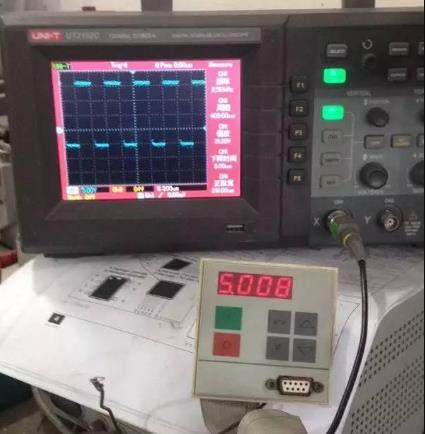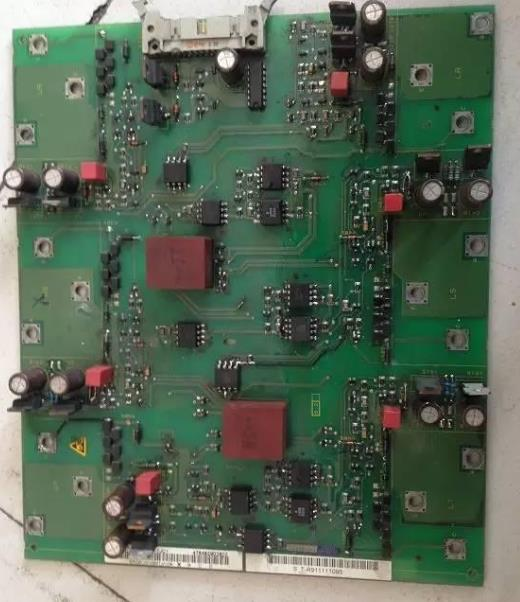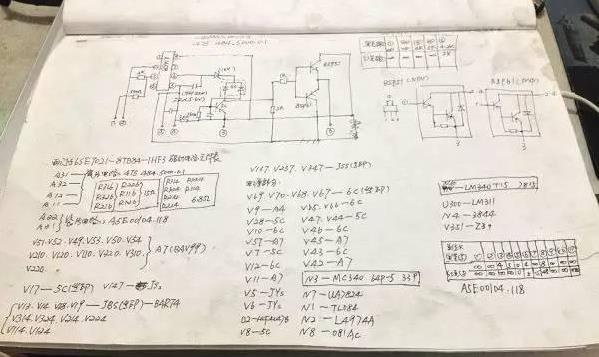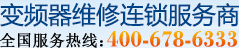17773132026

# 西门子6SE70变频器维修方法实例分析（太难得了！）

1.草图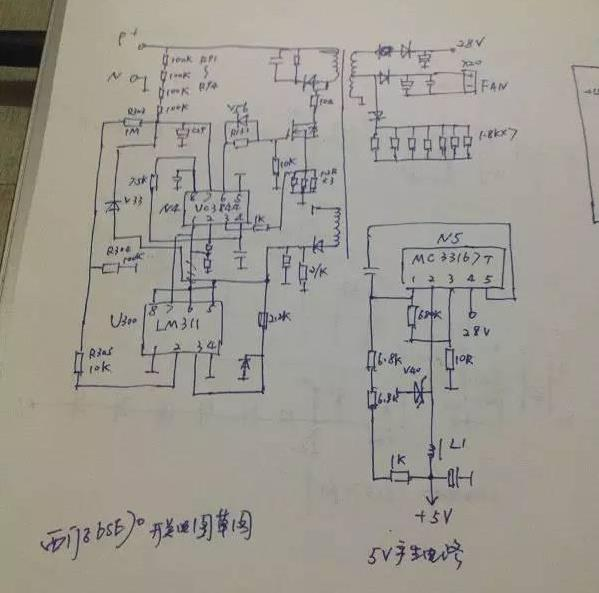2. 电流检测电路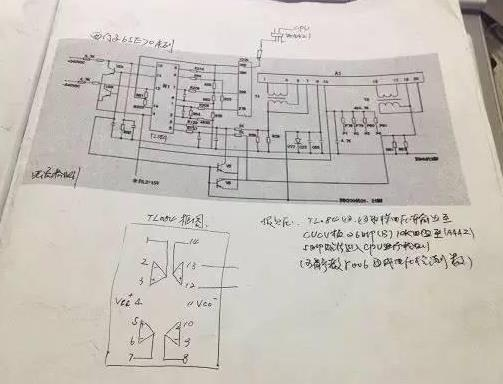11KW的70机采用T4 T5作为电流检测互感器，A1为电流检测陶瓷片上面有2个运放电路，11KW的电压检测也在这里。

22KW 上电就报F011

1=3.9V 2=4V 3=空脚 4=4V 5=4V 6=0V 7=0V 8=0.27V 9=-15V 10=+15V 11=0V 12=4V 13=0V 14=0V 15=4V 16=4V 17=4V 18=0V 19=4V 20=0V 。

3.22KW电压检测电路，此电路比较简单，采用运放082检测。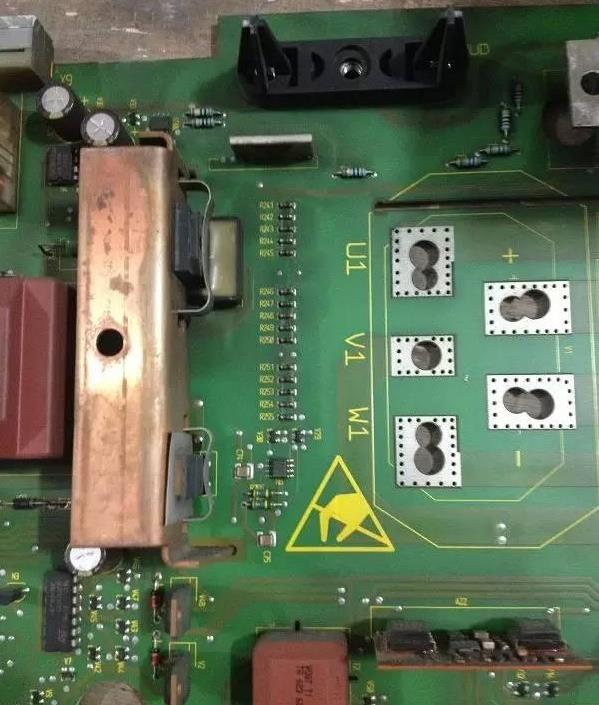4.驱动电路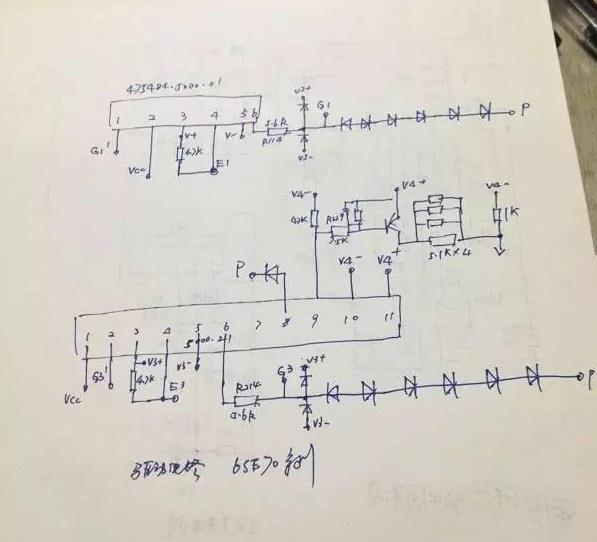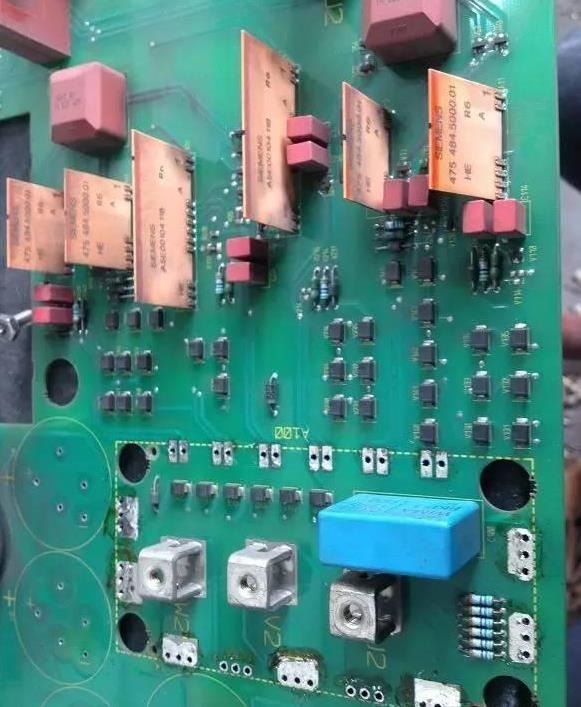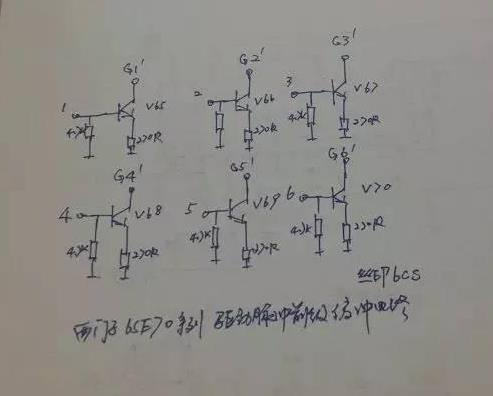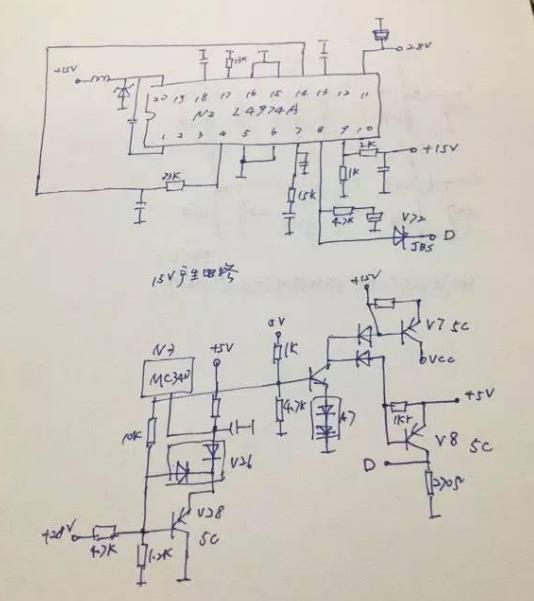L4974第11脚工作组电压由开关电源提供，测试其11脚电压正常，20脚输出为0V，其他各脚均不正常，由于没有时间分析L4974的工作原理，所以就查其外围元件，发现V8已经击穿，更换后工作正常。

L4974正常工作电压：

1=26.7V 2=3.1V 3=15V 4=5.1V 5,6,15,16=0V(接地） 7=4V 8=3.85V 9=5V 10=0.8V 11=27.7V 12=0.45V 13=5V 14=12V 17=10.5V 18=2.3V 19=0.3V 20=15V